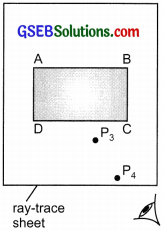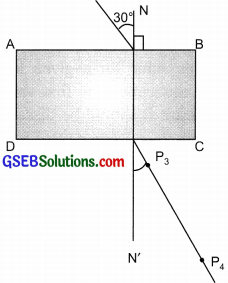# GSEB Solutions Class 10 Science Chapter 10 Light Reflection and Refraction

Gujarat Board GSEB Textbook Solutions Class 10 Science Chapter 10 Light Reflection and Refraction Textbook Questions and Answers, Additional Important Questions, Notes Pdf.

## Gujarat Board Textbook Solutions Class 10 Science Chapter 10 Light Reflection and Refraction

### Gujarat Board Class 10 Science Light Reflection and Refraction InText Questions and Answers

Question 1.
Define the principal focus of a concave mirror.
It is a point on the principal axis where the rays of light parallel to principal axis meet.

Question 2.
The radius of curvature of a spherical mirror is 20 cm. What is its focal length?
R = 20 cm
R = 2f
f = $$\frac {R}{2}$$, f =$$\frac {20}{2}$$ = 10 cm.
The focal length is 10 cm.

Question 3.
Name a mirror that can give an erect and enlarged image of an object.
Concave mirror.

Question 4.
Why do we prefer a convex mirror as a rear-view mirror in vehicles?
The convex mirror can cover a wider range and give the erect and diminished image. Hence convex mirror is used as a rear-view mirror to get a wider field of view.Question 5.
Find the focal length of a convex mirror whose radius of curvature is 32 cm.
R = 32 cm
R = 2f
f = $$\frac {R}{2}$$ = $$\frac {32}{2}$$ = 16 cm.

Question 6.
Placed at 10 cm in front of it. Where is the image located?
Object distance u = -10 cm (concave mirror)
Magnification m = -3
m = $$\frac {-v}{u}$$
v = – mu = -(-3) (-10) = -30 cm.
The image formed v = 30 cm in front of the concave mirror.

Question 7.
A ray of light traveling in air enters obliquely into water. Does the light ray bend towards the normal or away from the normal? Why?
The light bends towards the normal on entry into the water because water is optically denser than air.

Question 8.
Light enters from air to glass having a refractive index 1.50. What is the speed of light in the glass? The speed of light in a vacuum is 3 x 108 m/s.
The speed of light in vacuum is = 3 x 108 m/s
Refractive index of glass ng = 1.50
ng = $$\frac {c}{v}$$
Speed of light m glass Vg = $$\frac{c}{n_{g}}$$ = $$\frac{3 \times 10^{8}}{1.50}$$ = 2 x 108m/s.

Question 9.
Find out from the following table the medium having highest optical density Also find the medium with lowest optical density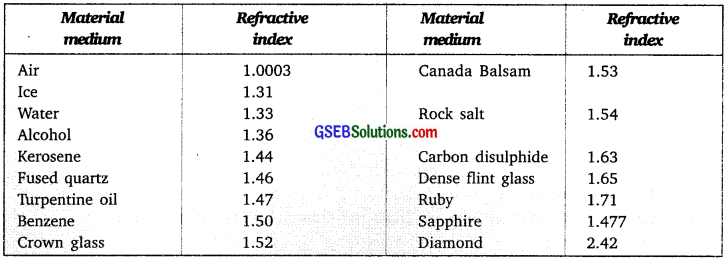Diamond has the highest optical density te., 2.42 and air has the lowest optical density
i.e., 1.0003.Question 10.
You are given kerosene, turpentine, and water. In which of these does the light travel fastest? Use the information given in the table above.
Refractive index of kerosene = 1.44
Refractive index of turpentine = 1.47
Refractive index of water = 1.33
Lower the refractive index faster is the speed of light in that medium. Hence light will travel fastest in water.

Question 11.
The refractive index of diamond is 2.42. What is the meaning of this statement?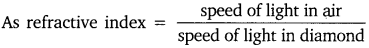This means the ratio of the speed of light in the air and the speed of light in the diamond is equal to 2.42.

Question 12.
Define 1 dioptre of power of a lens.
I dioptre is the power of a lens whose focal length is 1 meter.
1D = 1m-1

Question 13.
A convex lens forms a real and inverted image of a needle at a distance of 50 cm from it. Where is the needle placed in front of the convex lens if the image is equal to the size of the object? Also, find the power of the lens.
$$\frac {1}{v}$$ – $$\frac {1}{u}$$ = $$\frac {1}{f}$$
v = + 50 cm. Convex lens as image is of same size (i.e., m = -1) hence [ ∴ m = $$\frac {v}{u}$$]
u = -50 cm
$$\frac {1}{f}$$ = $$\frac {1}{(50 cm)}$$ – $$\frac {1}{(-50 cm)}$$ = $$\frac {1}{50}$$ + $$\frac {1}{50}$$ = $$\frac {1}{25}$$ cm
f = + 25cm =+ 0.25m.

Question 14.
Find the power of a concave lens of focal length 2 m.
Focal length of concave lens f = -2 m
Power of concave lens p =
p = $$\frac {1}{f}$$
p = $$\frac {1}{-2}$$ = -0.5 DIn-Text Activities Solved

Activity 10.1

• Take a large shining spoon. Try to view your face on its curved surface.
• The image obtained is larger.
• Move the spoon slowly away from your face.
• The image becomes inverted.
• Reverse the spoon and repeat the activity
• The image is diminished and virtual.
• object image object
• Enlarged image Diminished image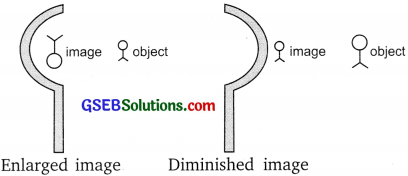Activity 10.2

• Hold a concave mirror in your hand and direct its reflecting surface towards the Sun.
• Direct the light reflected by the mirror on to a sheet of paper held close to the mirror.
• Move the sheet of paper back and forth gradually until you find on the paper
• sheet a bright, sharp spot of light.
• Hold the mirror and the paper in the same position for a few minutes.

Observation: The paper initially turns blackish, burns to produce smoke. Eventually, it catches fire.
Conclusion: The light from the sun is converged at a point on the paper. This point is the focus of the concave mirror.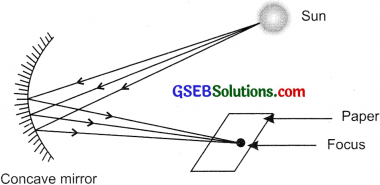Activity 10.3

• Take a concave mirror. Find out its approximate focal length. Left = 10 cm.
• Mark a line on the table with chalk. Place the concave mirror on a stand. Place the
• stand over the line such that its pole lies over the line.
• Draw with chalk two more lines parallel to the previous line such that the distance between two successive lines is equal to the focal length of the mirror.
• These lines will correspond to the positions of the points RF and C respectively.
• Keep a bright object say a burning candle, at a position far beyond C. Place a
• paper screen and move it in front of the mirror till you obtain a sharp bright image of the candle flame on it.
• Observe the image carefully. Note down its natural position and relative size with respect to the object size.
• Repeat the activity, by placing the candle –
(a) just beyond C
(b) at C
(c) between F and C
(d) at F
(e) between P and E
• In one of the cases, the image is not obtained on the screen i.e., when the object is between P and E

Observation:
Observation Table
image formation by a concave mirror: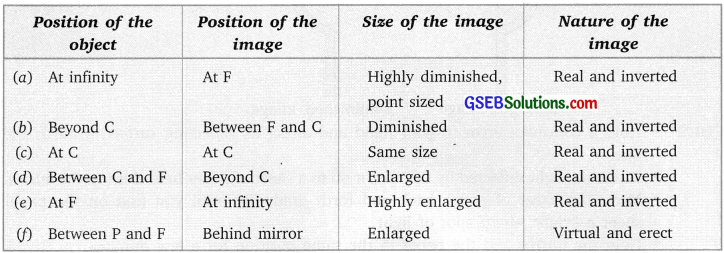Activity 10.4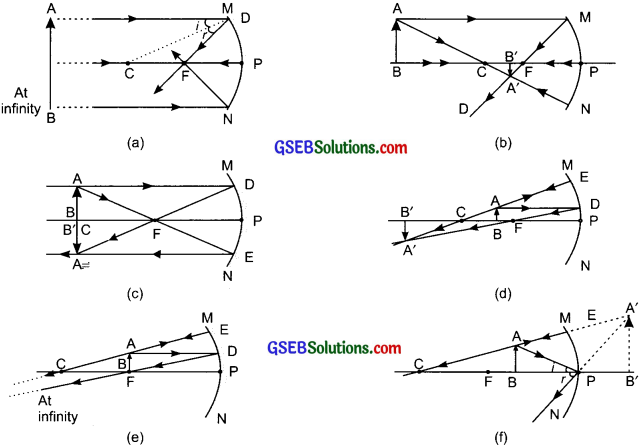Activity 10.5

• Take a convex mirror. Hold it in one hand.
• Hold a pencil in the upright position in the other hand.
• Observe the image of the pencil in the mirror.
Observation: The image is erect, diminished, and virtual.
• Move the pencil away from the mirror slowly.
Observation: The image formed is smaller and erect.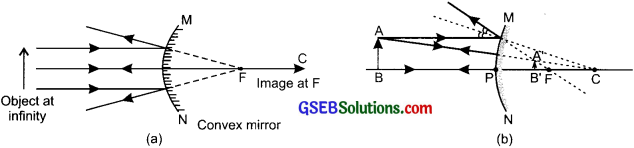Activity 10.6

• Observe the image of a distant object, say a distant tree, in a plane mirror.
Observation: The full length is not seen in a small plane mirror.
• Now use a concave mirror to see the full-length image of the object.
Observation: The full-length image is not obtained in a concave mirror.
• Now use a convex mirror to see the same.
Observation: In this case, a full-length image of an object is obtained in a small mirror.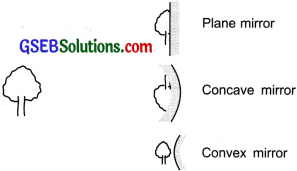Activity 10.7

• Place a coin at the bottom of a bucket filled with water.
• With your eye to a side above water, try to pick up the coin in one go.
Observation: One cannot pick up the coin in one go, because of refraction of light, the coin does not appear to be at its original position.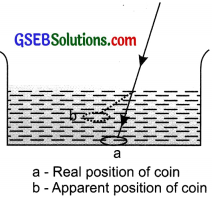Activity 10.8

• Place a large shallow bowl on a table and put a coin in it.
• Move away slowly from the bowl. Stop when the coin just disappears from your sight.
• Ask a friend to pour water gently into the bowl without disturbing the coin.
• Keep looking for the coin from your position.

Observation: The coin becomes visible again and slightly raised above its actual position on pouring water into the bowl. This is because of the refraction of light.Activity 10.9

• Draw a thick straight line in ink, over a sheet of white paper placed on a table.
• Place a glass slab over the line in such a way that one of its edges makes an angle with the line.
• Look at the portion of the line under the slab from the sides. The line appears to be bent at the edges.
• Now, place the glass slab such that it is normal to the line. The part of the line under the glass slab appears to be bent.
• Look at the line from the top of the glass slab. The line appears to be raised. It is due to the refraction of light.

Activity 10.10

• Fix a sheet of white paper on a drawing board using drawing pins.
• Place a rectangular glass slab over the sheet in the middle.
• Draw the outline of the slab with a pencil. Let us name the outline as ABCD.
• Take four identical pins.
• Fix two pins, E and F vertically such that the line joining the pins is inclined to the edge AB.
• Look for the images of the pins E and F through the opposite edge. Fix two pins, say G and H, such that these pins and the images of E and F lie on a straight line.
• Remove the pins and the slab.
• Join the positions of the tip of the pins E and F and produce the line up to AB. Let EF meet AB at O. Similarly, join the positions of the tip of the pins G and H and produce it up to the edge CD. Let HG meet CD at O’.
• Join O and O’. Also, produce EF up to I as shown by a dotted line.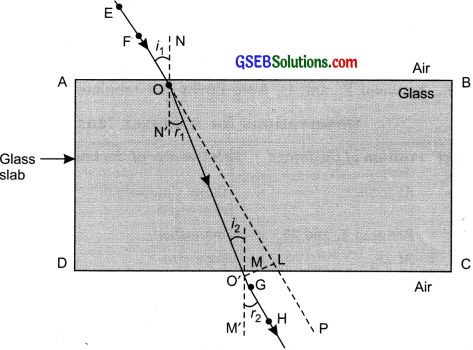Activity 10.11

• Hold a convex lens in your hand. Direct it towards the Sun. (Do not look at the
• Sun through the lens, or else it may cause permanent damage to eye).
• Focus the light from the Sun on a sheet of paper. Obtain a sharp bright image of the Sun.
• Hold the paper and the lens in the same position for a while to keep observing the paper.

Observation: The paper begins to burn to produce smoke, it may catch fire after a while.
Condition: The light rays from the sun get converged when it passes through the lens and a bright spot is formed on the paper, which is the focus of the Lens.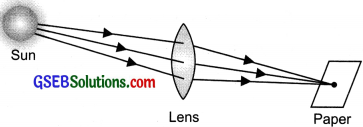Activity 10.12

• Take a convex lens. Find its approximate focal length in a way described in activity 10.11.
• Draw five parallel straight lines, using chalk, on a long Table such that the distance between the successive lines is equal to the focal length of the lens.
• Place the lens on a lens stand. Place it on the central line such that the optic center of the lens lies just over the line.
• The two lines on either side of the lens corresponding to F and 2F of the le respectively. Mark them with appropriate letters such as 2F1, F1, F2, and 2 respectively.
• Place a burning candle, far beyond 2F1 to the left. Obtain a clear sharp image a screen on the opposite side of the lens.
• Note down the nature, position, and relative size of the image.
• Repeat this activity by placing an object just behind 2F1. At 2F1, between F1 at 2F1 at F1, between F1 and O. Note down and tabulate the observations.

Observations for a convex lens: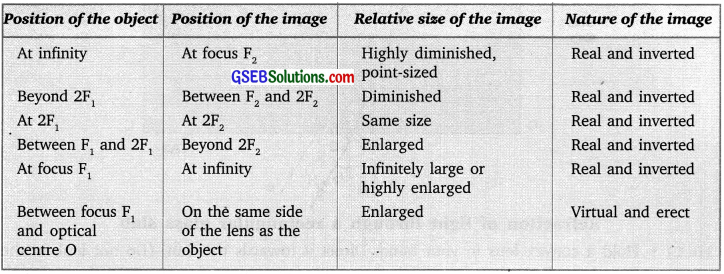Activity 10.13

• Take a concave lens. Place it on a lens stand.
• Place a burning candle on one side of the lens.
• Look through the lens from the other side and observe the image.
Try to get the image on a screen, if possible or else observe the image direc through the lens.
Note down the nature, relative size and approximate position of the image.
• Move the candle away from the lens.
• Note the change in the size of the image. Record your observations by placin candle at position too far away from the lens.

Observations for a concave lens: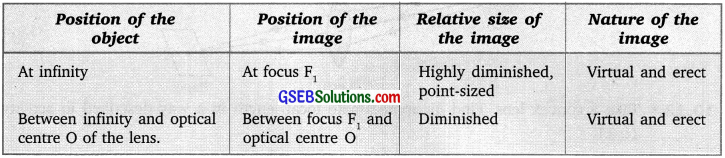### Gujarat Board Class 10 Science Light Reflection and Refraction Textbook Questions and Answers

Question 1.
Which one of the following materials cannot be used to make a lens?
(a) Water
(b) Glass
(C) Plastic
(d) Clay
(d) Clay

Question 2.
The image formed by a concave mirror is observed to be virtual, erect, and larger than the object. Where should be the position of the object?
(a) Between the principal focus and the center of curvature
(b) At the center of curvature
(c) Beyond the center of curvature
(d) Between the pole of the mirror and its principal focus
(d) Between the pole of the mirror and its principal focus.

Question 3.
Where should an object be placed in front of a convex lens to get a real image of the size of the object?
(a) At the principal focus of the lens
(b) At twice the focal length
(c) At infinity
(d) Between the optical center of the lens and its principal focus
(b) At twice the focal length.Question 4.
A spherical mirror and a thin spherical lens have each a focal length of -15 cm. The mirror and the lens are likely to be
(a) both concave
(b) both convex
(c) the mirror is concave and the lens in convex.
(d) the mirror is convex but the lens is concave.
(a) Both concave.

Question 5.
No matter how far you stand from a mirror, your image appears erect. The minor is likely to be
(a) plane
(b) concave.
(c) convex
(d) either plane or convex.
(d) either plane or convex.

Question 6.
Which of the following lenses would you prefer to use while reading small letters found in a dictionary?
(a) A convex lens of focal length 50 cm.
(b) A concave lens of focal length 50 cm.
(c) A convex lens of focal length 5 cm.
(d) A concave lens of focal length 5 cm.
(c) A convex lens of focal length 5 cm.Question 7.
We wish to obtain an erect image of an object, using a concave mirror of focal length 15 cm. What should be the range of distance of the object from the mirror? What is the nature of the image? Is the image larger or smaller than the object? Draw a ray diagram to show the image formation in this case.
The focal length of the concave mirror = 15 cm.
The object should be placed in front of the given concave mirror at a distance of less than 15 cm.
The image formed is virtual and erect.
Image size is larger than the object.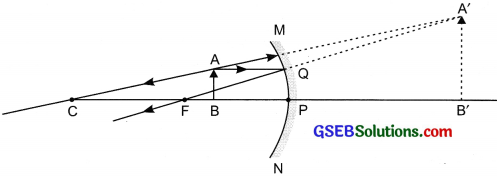Question 8.
Name the type of mirror used in the following situations.
(a) The headlight of a car.
(b) Side/rear-view mirror of a vehicle
(c) Solar furnace.
(a) For headlight of a car – The concave mirror is used to get a powerful beam of light after reflection.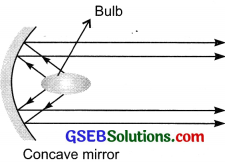(b) A convex mirror is used for side/rearview mirror of a vehicle. The convex mirror forms an erect and diminished image of vehicles and gives a wider view of the rear.
(c) In solar furnace concave mirror is used as a reflector, it concentrates sunlight at a point where the temperature increases sharply to 180°C – 200°C.Question 9.
One-half of a convex lens is covered with a black paper. Will this lens produce a complete image of the object? Verify your answer experimentally? Explain your observations.
Yes, one-half of a convex lens when covered with a black paper, the lens produces a complete or full image of an object.

To verify experimentally:
Take a convex lens, cover half part of it as shown in the figure, with a paper. Place it on a stand. Focus a distant object on a screen, the image obtained on the screen is complete.

Observation and conclusion:
Image formed on the screen does not depend on the size of the lens. The brightness of the image decreases as less number of rays pass through the lens.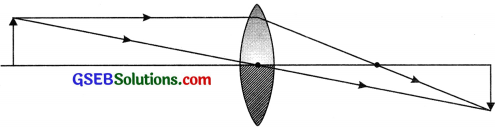Question 10.
An object 5 cm in length is held 25 cm away from a converging lens of focal length 10 cm. Draw the ray diagram and find the position, size, and nature of the image formed.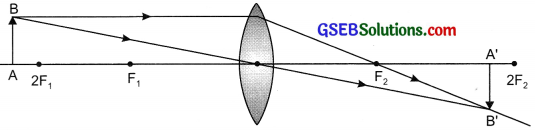f = 10cm
u = – 25 cm
h0 = 5 cm
Convex lens
$$\frac {1}{f}$$ = $$\frac {1}{v}$$ – $$\frac {1}{u}$$
$$\frac {1}{v}$$ = $$\frac {1}{f}$$ + $$\frac {1}{u}$$ = $$\frac {1}{10}$$ + $$\frac {1}{(-25)}$$ = $$\frac {5 – 2}{50}$$ = $$\frac {3}{50}$$
v = $$\frac {50}{3}$$ = 16.67 cm
Height of the image formed
$$\frac{h_{i}}{h_{o}}$$ = $$\frac {v}{u}$$
$$\frac{h_{i}}{5}$$ = $$\frac {16.67}{-25}$$
h1 = – 3.33 cm
Hence, the image formed at 16.67 cm from the lens on the other side. The size of the image is 3.33 cm, i.e., reduced and inverted.Question 11.
A concave lens of focal length 15 cm forms an image 10 cm from the lens. How far is the object placed from the lens? Draw the ray diagram.
Concave lens
f = -15 cm
v = – 10 cm
$$\frac {1}{v}$$ – $$\frac {1}{u}$$ = $$\frac {1}{f}$$
$$\frac {1}{-10}$$ – $$\frac {1}{u}$$ = $$\frac {1}{-15}$$
$$\frac {1}{u}$$ = – $$\frac {1}{30}$$ u = -30 cm
Ray diagram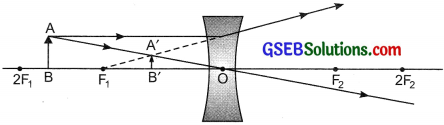Question 12.
An object is placed at a distance of 10 cm from a convex mirror of focal length 15 cm. Find the position and nature of the image.
Convex mirror
f = +15 cm, u = -10 cm.
$$\frac {1}{f}$$ = $$\frac {1}{v}$$ + $$\frac {1}{u}$$
$$\frac {1}{15}$$ = $$\frac {1}{v}$$ + $$\frac {1}{(-10)}$$
$$\frac {1}{v}$$ = $$\frac {1}{15}$$ + $$\frac {1}{10}$$
$$\frac {1}{v}$$ = $$\frac {5}{30}$$
v = + 60
The image is formed 6 cm behind the mirror, virtual image is formed.

Question 13.
The magnification produced by a plane mirror is +1. What does this mean?
Magnification, m = + 1.
+ indicates a virtual image.
1 indicates that the object size and image size is the same.Question 14.
An object 5.0 cm in length is placed at a distance of 20 cm in front of a convex mirror of radius of curvature 30 cm. Find the position of the image, its nature, and size.
f = $$\frac {R}{2}$$ = $$\frac {30}{2}$$ = 15cm
f = + 15 cm, u = – 20 cm, h = 5.0 cm
$$\frac {1}{v}$$ + $$\frac {1}{u}$$ = $$\frac {1}{f}$$
$$\frac {1}{v}$$ + $$\frac {1}{(-20)}$$ = $$\frac {1}{15}$$
$$\frac {1}{v}$$ = $$\frac {1}{15}$$ + $$\frac {1}{20}$$
$$\frac {1}{v}$$ = $$\frac {7}{20}$$
v = $$\frac {60}{7}$$ = 8.57 cm
The image is formed behind the mirror at a distance of 8.6 cm.
m = $$\frac{h_{2}}{h_{1}}$$ = – $$\frac {v}{u}$$
$$\frac{h_{2}}{5 \mathrm{cm}}$$ = $$\frac {-8.57}{(-20)}$$
h2 = $$\frac{8.57 \times 5 \mathrm{cm}}{20}$$
Height (size) of image = h2 = 2.14 cm
Thus, a 2.14 cm high, virtual and erect image is formed.

Question 15.
An object of size 7.0 cm is placed at 27 cm in front of a concave mirror of focal length 18 cm. At what distance from the mirror should a screen be placed so that a sharply focussed image can be obtained? Find the size and nature of the image.
Concave mirror u = – 27 cm, f = – 18 cm, h0 = 7.0 cm
Mirror formula
$$\frac {1}{v}$$ + $$\frac {1}{u}$$ = $$\frac {1}{f}$$
$$\frac {1}{v}$$ + $$\frac {1}{(-27)}$$ = $$\frac {1}{(18)}$$
$$\frac {1}{v}$$ = $$\frac {1}{v}$$ + $$\frac {1}{v}$$ = $$\frac {-3 + 2}{54}$$ = $$\frac { -1}{54}$$
v = -54 cm.
$$\frac{h_{2}}{h_{1}}$$ = – $$\frac {v}{u}$$
h1 = – $$\frac{v \times h_{o}}{u}$$ = $$\frac{-(-54) \times 7}{(-27)}$$ = – 14 cm
The image is real, inverted and enlarged.

Question 16.
Find the focal length of a lens of power – 2.0 D. What type of lens is this?
P = -2.0 D
p = $$\frac {1}{f}$$
f = $$\frac {1}{p}$$ = $$\frac {1}{-2.0 D}$$ = – 0.5m.
The lens is a concave lens as f = – ve.Question 17.
A doctor has prescribed a corrective lens of power + 1.5 D. Find the focal length of the lens. Is the prescribed lens diverging or converging?
P = + 1.5D,
P = $$\frac {1}{f}$$
Focal length of the lens f =$$\frac {1}{p}$$ = $$\frac {1}{+ 1.5 D}$$ = + 0.67 m
The power of the lens is +ve, and it is a converging lens i.e., convex lens

### Gujarat Board Class 10 Science Light Reflection and Refraction Additional Important Questions and Answers

Question 1.
What is light?
Light is a form of electromagnetic radiation that causes the sensation of sight. It doesn’t require any material medium to travel.

Question 2.
Name some phenomena associated with light during image formation by mirrors.
Reflection.

Question 3.
Define reflection of light.
The phenomenon of coming back of light in the same medium after striking a plane and polished surface is called reflection of light.

Question 4.
Define incident ray, reflected ray, normal ray, angle of incidence, and reflection.

• Incident ray – light that falls on the mirror/ polished surface is called incident ray. Reflected ray – ray of light that goes back in the same medium after striking the surface is called reflected ray.
• Normal – the perpendicular drawn to the reflecting surface is called normal at that point. The angle of incidence – the angle between the incident ray and the normal is known angle of incidence.
• The angle of reflection – the angle between the reflected ray and the normal is a known angle of reflection.

Question 5.
State laws of reflection. (CBSE 2011)
1. The incident ray, reflected ray and normal at the point of incidence all lie in the same plane.
2. The angle of incidence is equal to the angle of reflection.Question 6.
What are the properties of an image formed by a plane mirror?

• The image is virtual and erect.
• Size of the image is equal to that of object
• The image is laterally inverted.
• The image formed by a plane mirror is always at the same distance as the object is in front of it.

Question 7.
What are spherical mirrors?
Mirrors whose reflecting surface is part of a sphere are called spherical mirrors.

Question 8.
Define pole, the center of curvature, the radius of curvature, principal axis, aperture, focus, and focal length of a spherical mirror.
1. Pole: the center of reflecting surface. It is represented by letter P
2. Centre of Curvature: The center of the sphere of which the mirror forms the part. Represented by “C”.
3. The radius of Curvature: The radius of the sphere of which the mirror forms the part. Represented by “R”. meet (convex mirror) after reflection. Represented by F.
4. Focal Length: The distance between the pole and the principal focus of a spherical mirror is called the focal length. Represented by fQuestion 9.
Give some uses of a concave mirror.
(a) Used in torches, searchlights, and vehicle headlights.
(b) Used as a shaving mirror.
(c) Used by the dentist.
(d) Used in a solar furnace.

Question 10.
Give uses of a convex mirror.
(a) Used as a rearview mirror in vehicles.
(b) Used to see a full-length image of a falling building.

Question 11.
Give the sign conventions for spherical mirrors.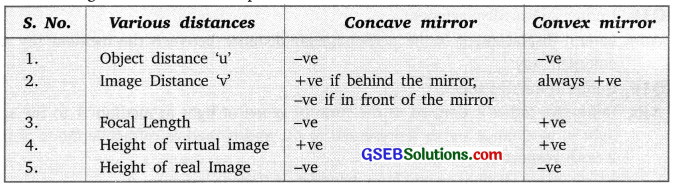Question 12.
State mirror formula and write it mathematically.
The relation between focal length of mirror, distance of the object and distance of the image is known as mirror formula. It is given by
$$\frac {1}{u}$$ + $$\frac {1}{v}$$ = $$\frac {1}{f}$$
v = Image distance
u = Object distance
f = Focal length

Question 13.
Give the relation between focal length and radius of curvature.
f = $$\frac {R}{2}$$

Question 14.
Define the magnification of the mirror.
The ratio of the height of the image to the height of the object is called magnification. It is represented by’
m = Height of image (h’)/Height of object (h) = $$\frac {-v}{u}$$
Magnification of real image is negative and of the virtual image is positive.

Question 15.
Define the refraction of light.
The change in direction of light, when it travels from one medium to another medium is called refraction of light.Question 16.
State laws of refraction.
The ratio of the sin of the angle of incidence to the sin of the angle of refraction for a light of given color and for a given pair of media is constant. This is called Snell’s law.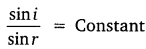The incident ray, refracted ray and the normal at the point of incidence lie on the same plane.

Question 17.
What do you observe when a light ray passes through a rectangular slab?
(a) The angle of incidence is equal to the angle of emergence.
(b) The incident ray is parallel to the emergent ray.
(c) Lateral displacement is proportional to the thickness of the glass slab.
(d) Lateral displacement is proportional to the angle of incidence.

Question 18.
Define lateral displacement.
Lateral displacement is the perpendicular distance between the incident ray and the emergent ray.

Question 19.
Define the refractive index.
The Refractive index is defined as the ratio of the speed of light in medium 1 to the speed of light in medium 2 and is represented as n21and is read as a refractive index of medium 2 with respect to medium 1.
n21 = speed of light in medium 1/speed of light in medium 2.

Question 20.
Define the absolute refractive index.
When medium 1 is a vacuum, then the refractive index of medium 2 is considered with respect to vacuum. This is called an absolute refractive index.Question 21.
What is the unit of the refractive index?
It has no unit.

Question 22.
Define optical density.
The ability of the medium to refract light is called optical density.

Question 23.
What is the relation between optical density, refractive index, and speed of light?
The medium with a higher refractive index in which speed of light is less is known as optically denser medium and the medium with lower refractive index in which the speed of light is more is known as an optically rarer medium.

Question 24.
State lens formula and write it mathematically.
The relationship between object distance (u), image distance (v), and focal length (f) of the lens is known as lens formula.
It is given by –
$$\frac {1}{v}$$ – $$\frac {1}{u}$$ = $$\frac {1}{f}$$

Question 25.
Define the magnification of the lens.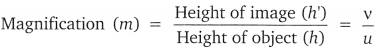For convex lens, ‘ni’ can be more than, less than or equal to one. For the concave lens, ‘rn’ is less than one.

Question 26.
Define a lens.
A transparent material bounded by two surfaces of which one or both surfaces are spherical forms a lens.

Question 27.
What are the two types of lenses?
Spherical lens: a combination of two spherical refracting surfaces.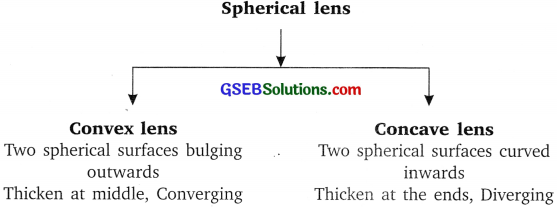Question 28.
Write the nature, position, and relative size of the image formed by a convex lens.
Table: Nature, position and relative size of the image formed by a convex lens for various positions of the object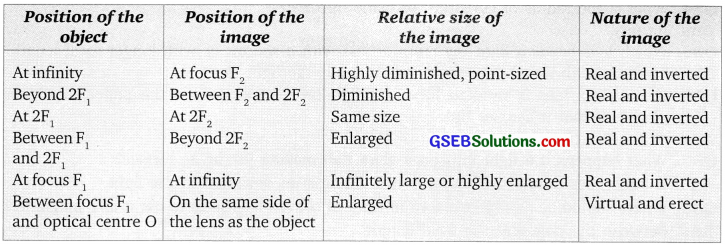Question 29.
Write the nature, position and relative size of the image formed by a concave lens.
Table: Nature, position and relative size of the image formed by a concave lens for various positions of the object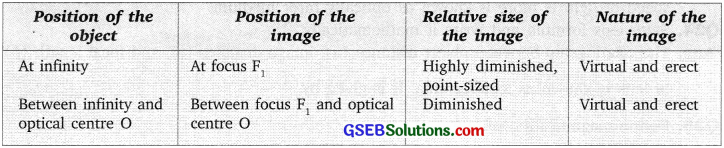Question 30.
Give sign conventions for spherical lenses.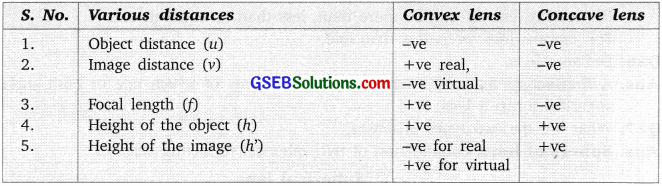Question 31.
Define the power of a lens.
The degree of convergence or divergence of light rays achieved by the lens is expressed in terms of power.
It is given by –
P = $$\frac {1}{f}$$
f = focal length in metreQuestion 32.
What is the S.l. unit of power? Define it.
The S.l. unît of power is dioptre denoted by “D”. 1 dioptre is the power of a lens whose focal length is 1 meter.
Power of a convex lens is + ve
Power of a concave lens – ve

Question 33.
What is the magnification of a plane mirror?
m = +1

Question 34.
What is the radius of the curvature of the plane mirror?
Infinity.

Question 35.
Which lens bends a light ray more or less with a shorter or with longer focal length?
The lens with the shorter focal length bends the light more.

Question 36.
If a convex lens is used to focus sunlight on a paper, where the paper should be placed so that it catches fire.
At the Principal focus.Question 37.
What happens if light falls on a glass slab making 900 at its surface?
It undergoes normal refraction that is there is no deviation in the light.

Question 38.
Where should be an object placed in front of the convex lens so as to use it as a magnifier?
Between the pole and the focal length.

Question 39.
What is the silvering of the mirror?
Silvering of mirror means coating the surface of the mirror with a thin layer of silver, aluminum, or some other shiny, opaque material.

Question 1.
State the laws of reflection of light.
Laws of reflection of light are:
1. The angle of incidence is equal to the angle of reflection and
2. The incident ray, the normal to the mirror at the point of incidence and the reflected ray, all lie in the same plane.

Question 2.
What are the properties of the image formed by a plane mirror?
The image formed by a plane mirror is always virtual and erect. The size of the image is equal to that of the object. The image formed is as far behind the mirror as the object is in front of it and the image is laterally inverted.

Question 3.
Define pole and center of curvature of spherical mirrors.
Pole: The center of the reflecting surface of a spherical mirror is a point called the pole. It lies on the surface of the mirror. It is represented by “P”. Centre of Curvature: The reflecting surface of a spherical mirror forms a part of a sphere, this sphere has a center and this point is called the center of curvature of the spherical mirror.Question 4.
Give the uses of concave mirrors.

1. Concave mirrors are used in torches, search-lights, and vehicle headlights to get powerful parallel beams of light.
2. They are also used as shaving mirrors to see a large image of the face.
3. Dentists use concave mirrors to see large images of the teeth of patients.
4. Large concave mirrors are used in making solar furnaces, solar cookers, etc.

Question 5.
Give the uses of convex mirrors.
Convex mirrors are commonly used as rear-view mirrors in vehicles. As these mirrors can give an erect image, wider field of view, these mirrors are used in vehicles to see the traffic behind.

Question 6.
Write the position, nature, and size of images formed by a concave mirror.
Table: Image formation by a concave mirror for different positions of the object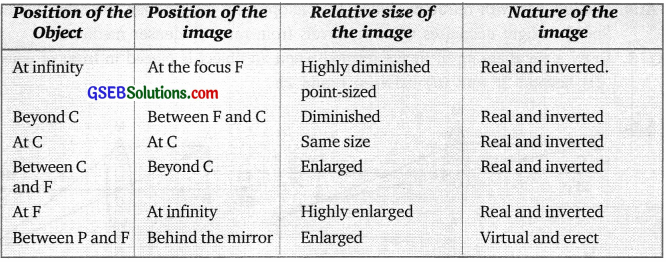Question 7.
Give the laws of refraction of light.
The laws of refraction of light are
1. The incident ray, the refracted ray, and the normal to the interface of two transparent media at the point of incidence all lie in the same plane.
2. The ratio of the sine of the angle of incidence to the sine of the angle of refraction is constant, for the light of a given color and for the given pair of media. This law is also known as Snell’s law of refraction.Question 8.
What is the refractive index?
If ‘i’ is the angle of incidence and ‘r’ is the angle of refraction then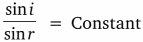This constant value is called the refractive index of the second medium with respect to the first.

Question 9.
What is the absolute refractive index of the medium?
When the refractive index of medium 2 is considered with respect to vacuum. This is called the absolute refractive index of the medium.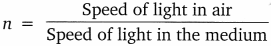Question 10.
Two medium with refractive index 1.31 and 1.50 are given. In which case
(1) bending of light is more?
(2) speed of light is more?
1. The bending of light is more in the medium where the refractive index is 1.50.
2. Speed of light is ‘more in the medium with refractive index 1.31

Question 11.
The Refractive index of kerosene oil is 1.44 and that of water is 1.33. A ray of light enters from kerosene oil to water. Where would’ light ray bend and why?
A ray of light enters from kerosene oil to water i.e., refractive index 1.44 to 1.33 i.e., from denser to rarer medium. Hence the ray of light bends away from the normal.Question 12.
Which is optically denser out of the two medium M1 = 1.71 (refractive index) and M2 = 1.36 (refractive index). How does speed of light change when it travels from optically rarer to denser medium.
Medium M1 with refractive index 1.71 is optically denser than the other medium M2. The speed of light decreases when it travels from rarer to denser medium.

Question 13.
Draw a ray diagram of the image formed when an object is placed in front of a convex lens
1. beyond 2F
2. between F and 2F.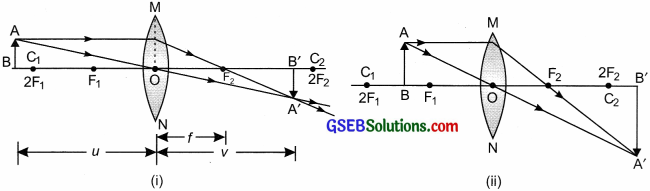Question 14.
Comment on the size, the position of the image formed by a concave mirror of focal length 18 cm when an object is placed:
1. at 22 cm
2. 14 cm
3.  40 cm.
in front of the mirror without calculations.

1. When the object is placed at 22 cm, the image is formed beyond 36 cm, real, inverted, image is magnified.
2. When the object is at 14 cm then the image formed is virtual and erect, behind the mirror and magnified.
3. When the object is placed beyond 40 cm, then the image is formed between 18 cm and 36 cm, it is a real, inverted, and diminished image.

Question 15.
Complete the following ray diagrams: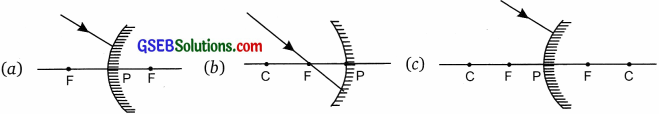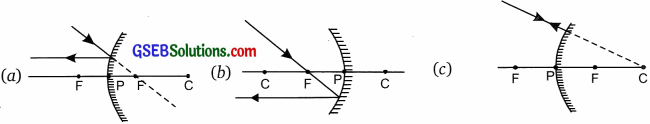Question 16.
With the help of a ray diagram showing how a pencil appears when dipped in water.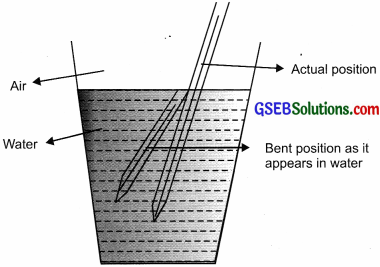A ray of light (as we see a pencil in air passing into water) travels from rarer to denser medium i.e., from air to water, it bends towards the normal, hence the pencil appears to be bent in water as shown in the diagram.

Question 17.
Define the power of the lens. What is the S. I. unit of power of a lens? 1f power of lens is + 2D what is the nature and focal length of the lens?
Power of lens: The degree of convergence or divergence of light rays obtained by a lens is expressed in terms of its power.
The power of a lens is defined as the reciprocal of its focal length.
p = $$\frac {1}{f}$$
S.l. unit of power of a lens is ‘dioptre’.
P = + 2D.
The lens is convex and the focal length of the lens is + 0.50 m.
(P = $$\frac {1}{f}$$, 2D = $$\frac {1}{f}$$,
∴ f = $$\frac {1}{2}$$= 0.5mQuestion 18.
If the speed of light in water is 2.25 x 108 m/s and the speed in a vacuum is 3 x 108m/s. Calculate the refractive index of water.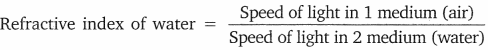∴ nw = $$\frac {c}{v}$$
nw = $$\frac{3 \times 10^{8}}{2.25 \times 10^{8}}$$
nw = 1.33
∴ The refractive index of water = 1.33.

Question 19.
The refractive index of water is 1.33 and kerosene is 1.44. Calculate the refractive index of kerosene with respect to water.
Refractive index of water = nw = 1.33
Refractive index of kerosene = nk = 1.44
∴ Refractive index of kerosene with respect to water is
nkw = $$\frac{n_{k}}{n_{w}}=\frac{1.44}{1.33}$$ = 1.08

Question 20.
Redraw the given diagram and show the path of the refracted ray.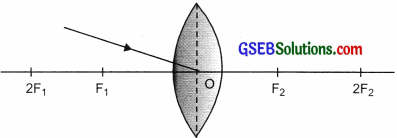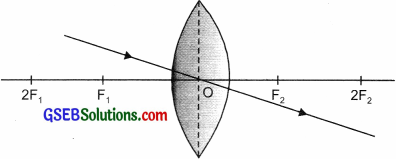Question 21.
Why does a ray of light bend when it travels from one medium into another?
Due to a change in velocity in the medium and to reduce the time taken to travel the same path, a ray of light bends when it travels from one medium to another.

Question 22.
Draw the given diagram in your answer book and complete it for the path of ray of light beyond the lens.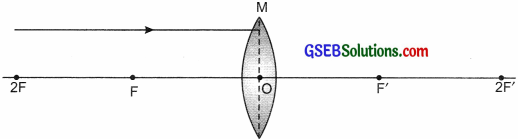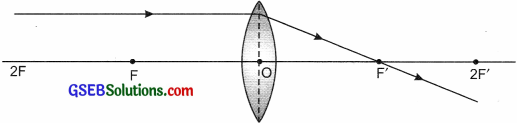Question 23.
What is the minimum number of rays required for locating the image formed by a concave mirror for an object? Draw a ray diagram to show the formation of a virtual image by a concave mirror.
Two rays: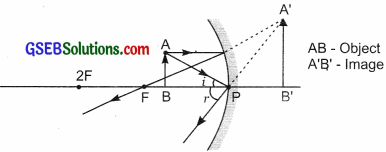Question 24.
Takedown this diagram on to your answer book and complete the path of the ray.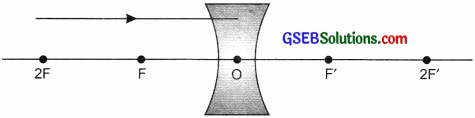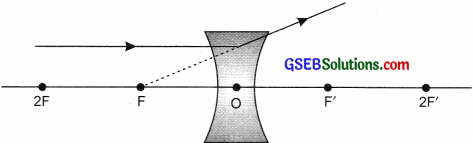Question 25.
What kind of mirrors are used in big shopping stores to watch the activities of customers?
Convex mirror as the image is independent of the position of the object.Question 26.
Draw a ray diagram to determine the position of the image formed of an object placed between the pole and the focus of a concave mirror.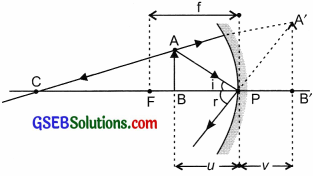Question 27.
State the mirror formula, lens formula and power of lens.
Mirror formula
$$\frac {1}{v}$$ + $$\frac {1}{u}$$ = $$\frac {1}{f}$$
(v = image distance, u = object distance, f = focal length)
Lens formula
$$\frac {1}{v}$$ – $$\frac {1}{u}$$ = $$\frac {1}{f}$$
Power of lens
P = $$\frac {1}{f}$$ f = focal length in metres

Question 28.
Draw ray diagrams to show the image formed by a concave lens for the object placed at
1. infinity
2. Between F and 2F of the lens.
1. object at infinity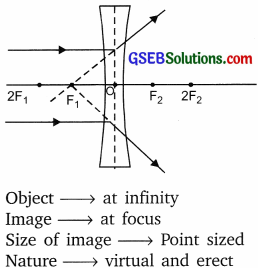2. the object between f and 2f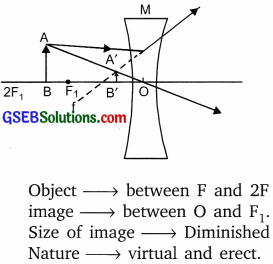Question 1.
With the help of a ray, a diagram showing the type of images formed when the object is at the following positions in front of a concave mirror.
(a) at infinity
(b) beyond C
(c) atF
(d) atC
(e) between F and C
(f) between F and P
C = centre of curvature
P = pole of the mirror
F = focus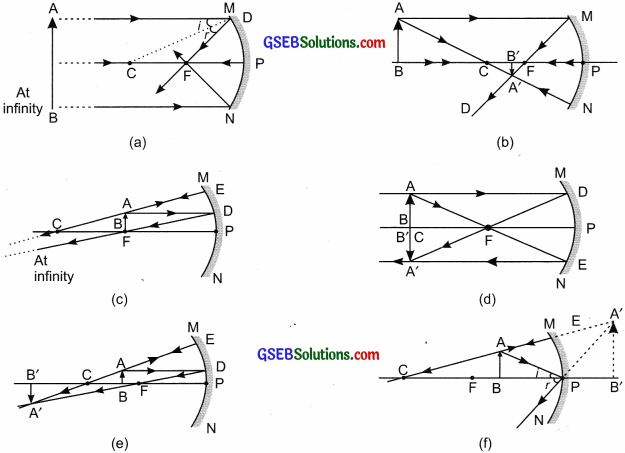Question 2.
With the help of a ray diagram show the position, size and the nature of the image formed by a convex lens for various positions of the object.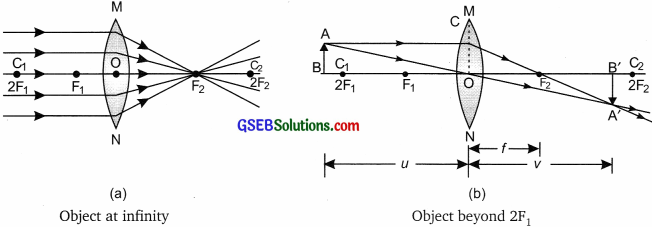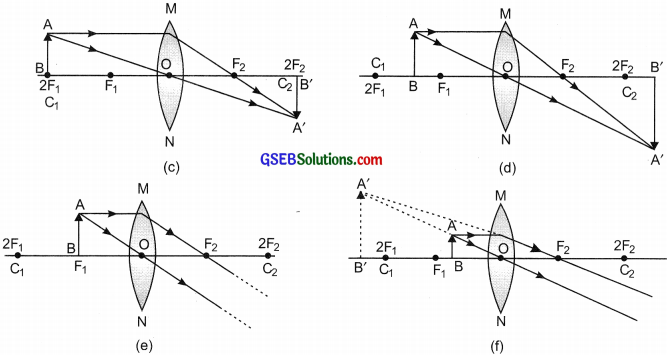Question 3.
Name the type of mirror used in the following situations:

1. Rearview mirror in vehicles
2. Solar furnace
3. Torch
4. Solar cooker
5. To get the full-length image of a tall building.

1. Rearview mirror in vehicles – convex mirror as it gives a virtual image, diminished, and cover the wider view.
2. Solar furnace – concave mirror to concentrate all parallel beam of light.
3. Torch – concave mirror is used.
4. Solar cooker – the concave mirror is used to concentrate .the heat rays at a point.
5. The convex mirror is used to view a full-length tall building.

Question 4.
Draw and explain the ray diagram formed by a convex mirror when
(a) the object is at infinity
(b) the object is a finite distance from the mirror.
(a) When the object is at infinity the image is formed at focus. ‘F’.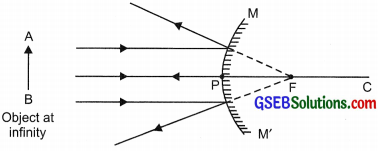(b) When the object is at a finite distance the image is formed behind the mirror, it is a virtual image and diminished in size.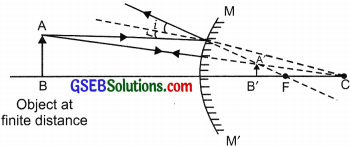Question 5.
A convex lens has a focal length of 15 cm. At what distance from the lens should the object be placed so that is forms on its other side a real and inverted image 30 cm away from the lens? What would be the size of the image formed if the object is 5 cm high? With the help of a ray, the diagram shows the formation of the image by the lens in this case.
f = 15cm
v = + 30 cm as image is real and inverted
$$\frac {1}{f}$$ = $$\frac {1}{v}$$ – $$\frac {1}{u}$$, we get
$$\frac {1}{u}$$ – $$\frac {1}{v}$$ = $$\frac {1}{f}$$ = $$\frac {1}{30}$$ – $$\frac {1}{15}$$ = $$\frac {1 – 2}{30}$$ = – $$\frac {1}{30}$$
u = – 30 cm (= 2f)
So, image should be of the same size as the object. Height of image = 5 cm.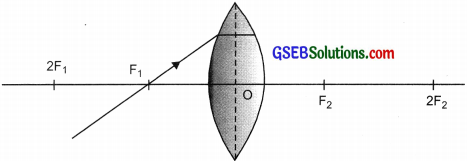Question 6.
Redraw the given diagram and show the path of the refracted ray.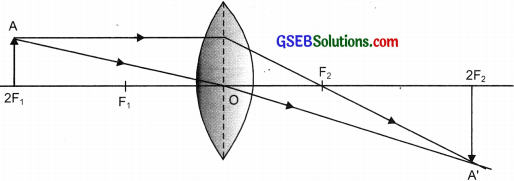A ray of light passing through a principal focus after refraction from a convex lens will emerge parallel to the principal axis.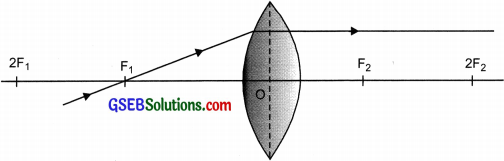Question 7.
A convex lens has a focal length of 12 cm. At what distance from the lens should an object of height 6 cm be placed so that on the other side of the lens its real and inverted image is formed 24 cm away from the lens? What would be the size of the image formed? Draw a ray diagram to show the image formed in this case.
f = + 12 cm
Real and inverted image so v = + 24 cm
$$\frac {1}{f}$$ = $$\frac {1}{v}$$ – $$\frac {1}{u}$$, we get
$$\frac {1}{u}$$ – $$\frac {1}{v}$$ = $$\frac {1}{f}$$ = $$\frac {1}{24}$$ – $$\frac {1}{12}$$ = $$\frac {1 – 2}{24}$$ = – $$\frac {1}{24}$$
u = -24 cm (= 2f)
So image will be of the same size as the object. Height of image = 6 cm.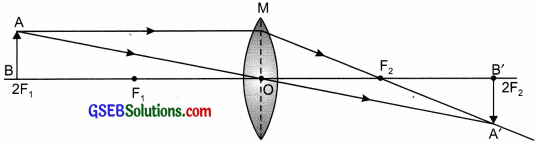Questions On Higher Order Thinking Skills (Hots)

Question 1.
Amit visited a fair and saw a mirror in which he got a very funny image. The above part of his body was big in size, the middle part was of normal size and the lower part of the body showed a very small size. What kind of mirror ¡s this?
Upper part – Concave mirror;
Middle part: Plane mirror;
Lower part: Convex mirror.Question 2.
Nidhi wanted the image of her pencil to be double the size of its original size. Name the mirror used for getting such an image.
Concave mirror.

Question 3.
Give the mirror image of ‘AMBULANCE”
DWAJUMA

Question 4.
An incident ray makes an angle of 600 with the mirror. What is the angle of reflection?
30°.

Question 5.
Define the following.
(a) What is a ray?
(b) What is the beam?
(c) What is the reflection of light?
(d) What is a reflector?
(e) What is the focal length?
(f) What is the principal focus?
(g) What is refraction?
(h) What is an optically rare medium?
(i) What is an optically denser medium?
(j) What is power?
(k) What is 1 dioptre?
(a) It is the path of light.
(b) Group of parallel light rays emitted by the source of light.
(c) Bouncing back of light after striking any surface.
(d) The surface reflects the light.
(e) The distance between the pole and the principal focus of the spherical mirror.
(f) A point of the principal axis where the rays of light parallel to principal axis meet.
(g) Bending of light rays when it travels from one medium to another.
(h) When the speed of light is more as compared to other mediums.
(i) When the speed of light is less as compared to another medium.
(j) The degree of convergence or divergence of light rays achieved by a lens is expressed in terms of its power
(k) It is the power of lens whose focal length is 1 m.

Question 6.
What are the two types of reflection?
(i) Regular
(ii) Irregular

Question 7.
Write the laws of reflection.
(a) The angle of incidence is equal to the angle of reflection.
(b) The incident ray, the normal to the mirror at the point of incidence and the reflected ray, all lie in the same plane.Question 8.
Give characteristics of the image formed by a plane mirror

• The image is virtual and erect.
• Size is the same as the object.
• It is formed at the same distance.

Question 9.
Give uses of a plane mirror.

• Looking glass
• Used in submarines
• Solar cooker
• Kaleidoscope

Question 10.
Name two types of spherical mirrors.
1. Concave mirror
2. Convex mirror

Question 11.
Give uses of a concave mirror.

• Used as reflectors in car headlights, searchlights, etc.
• Used as a shaving mirror.
• Used in solar cookers to focus the sunlight on one point.

Question 12.
Give uses of a convex mirror.

• It is used as the rearview mirror in cars.
• It is used in street lights as it diverges the light over a larger area.

Question 13.
What are the two types of refractive index?
Relative refractive index – It is the ratio of the speed of light in one medium to the speed of light in another medium
Absolute refractive index – It is the ratio of light in a vacuum to the speed of light in another medium.Question 14.
Why do we prefer a convex mirror as a rearview mirror in vehicles?
Convex mirrors are used as a rearview mirror in cars because it produces an erect and diminished image of the traffic behind the vehicle. It also gives a wider view.

Question 15.
Name the type of mirror used in the following situations.
(b) Side/rear-view mirror of a vehicle.
(c) Solar furnace.
(a) Concave mirror
(b) Convex mirror
(c) Concave mirror

Question 16.
The magnification produced by a plane mirror is +1. What does this mean?
This means that the size of the image is equal to the size of the object.Question 17.
Find the focal length of a lens of power – 2.0 D. What type of lens is this?
Given, Power = – 2.0
The given lens is concave (power of the concave lens is – ve)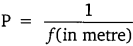-2.0 = $$\frac {1}{f}$$
f = $$\frac {1}{-2}$$ m
f = – 50 cm
The focal length is – 50 cm.

Practical Based Questions (Solved)

Question 1.
A student finds the focal length of the convex lens and records it as given below: 10 cm, 10.6 cm, 10.5 cm, and 10.5 cm. Which reading he should discard and why?
The focal length of the convex lens is taken as a distance between the lens and the image of the distant object. This value for a given lens is constant and hence the value of focal length should be the same. The first value taken as 10cm is not close to the other three values and hence it should be discarded. If the readings are taken carefully they should be the same.

Question 2.
Suggest how can one avoid the errors during measuring the focal length of the given lens.
The following errors can be caused and can be avoided:
(a) If the distant object is not taken the focal length may not be accurate.
(b) For finding the focal length keep the distant object the same and try to take at least
three readings, if they are same you have achieved the precision but if they are very close to each other with the difference of ± 0.1cm can be considered and the average of the same can be obtained.Question 3.
A student wants to find the refractive index of the glass slab given to him. What are the materials required to study the same in the lab? Give the formula to calculate the refractive index.
To find the refractive index of glass slab the student can use the glass slab, four-six pins, pencil, protractor, soft board, and a white sheet with four board pins. The formula for calculating the refractive index is:
n =Question 4.
Explain how practically you can identify the concave lens and convex lens in the lab. Justify your answer.
To identify the lenses we should try and get the real focus of the lens by trying to get the image of the distant object on the screen. The lens that gives the real focus is the convex lens and the one which does not give real focus is the concave lens. This is because the convex lens is a converging lens and all the rays passing through it converge to meet at one point called the focus. The concave lens is the diverging lens and the rays spread out and do not meet.

Question 5.
If you have to construct a microscope to view the minute objects and magnify them, suggest how will you do it practically and what is the material required for the same.